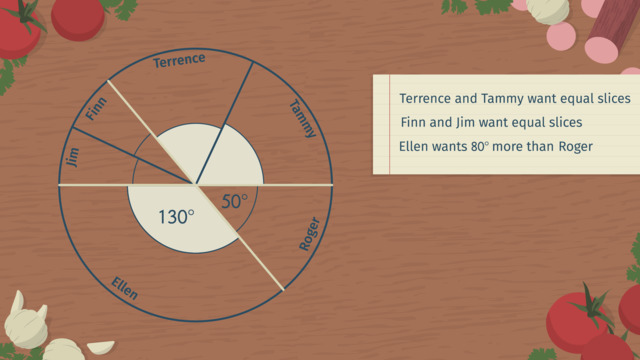# Calculations with Supplementary, Complementary, and Vertical AnglesRate this video

Ø 5.0 / 1 ratings

The authorChris S.

## Information about the videoCalculations with Supplementary, Complementary, and Vertical Angles

After this lesson you will be able to use definitions of special angles to solve for unknown angles.

The lesson begins by defining and identifying right, complementary, supplementary, and vertical angles. It leads to finding the measurements of angles given two measurements. It concludes with solving for angle measurements by using angle relationships to set up equations.

Learn about angles by helping Pete create the perfect pizza slices for his customers at Pete’s Pizza Paradise!

This video includes key concepts, notation, and vocabulary such as: adjacent angles (two angles who share a “side” (a ray) and a vertex); supplementary angles (two angles that sum to 180 degrees); complementary angles (two angles that sum to 90 degrees); vertical angles (“opposite” angles with equal measures that are formed by two intersecting); Vertical Angle Theorem (Vertical angles are congruent).

Before watching this video, you should already be familiar with the terms: adjacent, supplementary angles, complementary angles, vertical angles, and the relationships between them.

After watching this video, you will be prepared to learn about angle relationships when parallel lines are cut by a transversal.

Common Core Standard(s) in focus: 7.G.B.5 A video intended for math students in the 7th grade Recommended for students who are 12-13 years old